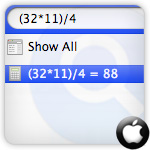# Use Spotlight For Quick Math Calculations on Mac OS X Snow Leopard [Time Save]

Although you may use the default calculator on Mac but for quick calculations Spotlight also includes calculator feature where users can directly enter math quires to get faster calculated results. Follow the simple instructions written below to know that how Spotlight works as calculator.Use Spotlight to perform mathematical calculations

Just type your mathematical query in Spotlight search field in right upper corner of your Mac screen, it quickly shows the results automaticallyYou can also perform Trigonometry, Exponential and Logarithm.

Trigonometry

cosinus cos(), tangent tan(), arc sinus asin(), arc cosinus acos(), arc tangent atan(),

Approximation

ceil(), rint()

Exponential and Logarithm

exp(), pow(), sqrt(), cbrt(), log()

for complete list of functions see the math libarary

Remi March 3, 2010, 1:57 am

Nice Spotlight feature.

Asst July 28, 2010, 11:17 pm

This is really nice to do some math quickly in spotlight search.